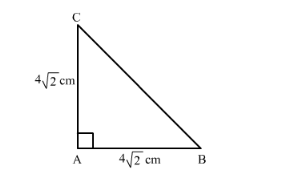# The length of the hypotenuse of an isosceles right triangle whose one side is 42 cm is

Question:

The length of the hypotenuse of an isosceles right triangle whose one side is 42 cm is

(a) 12 cm
(b) 8 cm
(c) 82 cm
(d) 122 cm

Solution:

Given: One side of isosceles right triangle is 4√2cm

To find: Length of the hypotenuse.

We know that in isosceles triangle two sides are equal.In isosceles right triangle $\mathrm{ABC}$, let $\mathrm{AB}$ and $\mathrm{AC}$ be the two equal sides of measure $4 \sqrt{2} \mathrm{~cm}$.

Applying Pythagoras theorem, we get

$\mathrm{BC}^{2}=\mathrm{AB}^{2}+\mathrm{AC}^{2}$

$\mathrm{BC}^{2}=(4 \sqrt{2})^{2}+(4 \sqrt{2})^{2}$

$\mathrm{BC}^{2}=32+32$

$\mathrm{BC}^{2}=64$

$B C=8 \mathrm{~cm}$